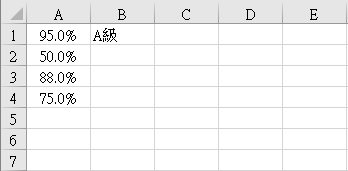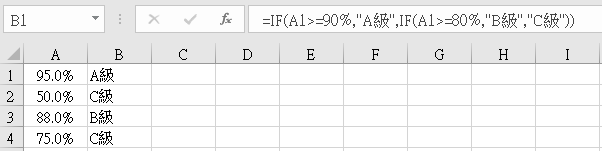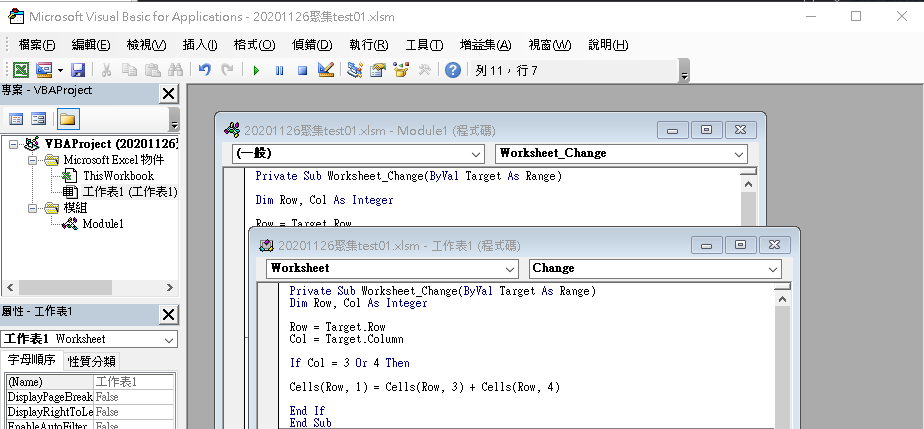#0

## [VBA]如何讓特定欄更改資料時，進行程度判別Private Sub Worksheet_Change(ByVal Target As Range)
Dim col, row As Integer
Dim i As Integer
col = Target.Column
row = Target.row
i = Target.Column + 1

If Cells(row, col) >= 0.9 Then Cells(row, i) = "A級"
ElseIf Cells(row, col) >= 0.8 Then Cells(row, i) = "B級"
Else: Cells(row, i) = "C級"
End If
End If

End Sub

### 1 個回答

0

``````Private Sub Worksheet_Change(ByVal Target As Excel.Range)
If Target.Column = 1 Then
...你要做的事情...
End If
End Sub
``````

MagarDane iT邦新手 5 級 ‧ 2020-11-27 20:46:07 檢舉Worksheet_Change 應該寫在那裡比較合理？

MagarDane iT邦新手 5 級 ‧ 2020-11-28 00:12:32 檢舉